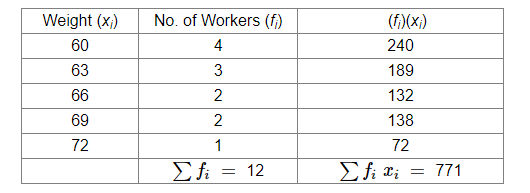# The following table shows the weights of 12 workers in a factory:

Question:

The following table shows the weights of 12 workers in a factory:Find the mean weight of the workers.

Solution:

We will make the following table:Thus, we have:

Mean $=\frac{\sum f_{i} x_{i}}{\sum x_{i}}$

$=\frac{771}{12}=64.25 \mathrm{~kg}$